Courses

# Test: Properties of Moist Air - 2

## 10 Questions MCQ Test Topicwise Question Bank for Mechanical Engineering | Test: Properties of Moist Air - 2

Description
This mock test of Test: Properties of Moist Air - 2 for Mechanical Engineering helps you for every Mechanical Engineering entrance exam. This contains 10 Multiple Choice Questions for Mechanical Engineering Test: Properties of Moist Air - 2 (mcq) to study with solutions a complete question bank. The solved questions answers in this Test: Properties of Moist Air - 2 quiz give you a good mix of easy questions and tough questions. Mechanical Engineering students definitely take this Test: Properties of Moist Air - 2 exercise for a better result in the exam. You can find other Test: Properties of Moist Air - 2 extra questions, long questions & short questions for Mechanical Engineering on EduRev as well by searching above.
QUESTION: 1

Solution:
QUESTION: 2

### If Pa and Pv denote respectively the partial pressure of dry air and that of water vapour in moist air, the specific humidity of air is given by

Solution:

Assuming that the dry air and water vapour behaves as perfect gases, we have for dryFor water vapour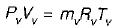Also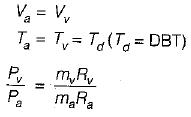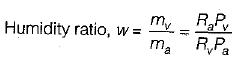Ra = 0.287 kJ/kgK (for dry air)
Rv = 0.461 kJ/kgK (for water vapour)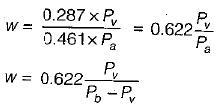QUESTION: 3

### The mass of water vapour in one m3 of dry air is 'defined as

Solution:

Mass of water vapour present per m3 of dry air is called absolute humidity.

QUESTION: 4

Indication of amount of moisture in air is given by

Solution:

The temperature measured by wick-covered bulb of a thermometer is called wet bulb temperature.
Such a thermometer or WBT indicates the temperature of liquid water in the wick.

QUESTION: 5

What is the saturation temperature at the partial pressure of water vapour in the air water-vapour mixture called

Solution:

Dew point temperature is the temperature of air recorded by a thermometer, when the moisture (water vapour) present in it begins condense.
In other words dew point temperature is the saturation temperature corresponding to the, partial pressure of water vapour.

QUESTION: 6

Choose the correct alternative

Solution: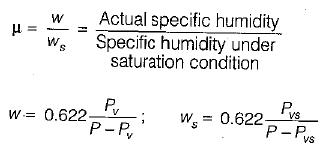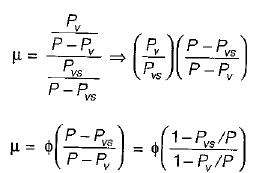QUESTION: 7

Dry bulb temperature, wet bulb temperature and dew-point temperature are same for which one of the following

Solution:

For saturated air, dry bulb temperature, wet bulb temperature and dew point temperature are same

QUESTION: 8

Which of the following option is true for the dry bulb temperature (TDBT), the wet bulb temperature (TWBT) and dew point temperature (TDPT) for a mixture of air and water vapour

Solution:

For a mixture of air and water vapour.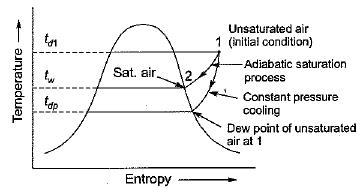QUESTION: 9

Degree of saturation or percentage humidity is

Solution:

Degree of saturation is the ratio of actual specific humidity to the specific humidity under saturated condition.

QUESTION: 10

For air at a given temperature as the relative humidity is increased isothermally

Solution: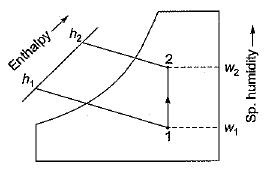WBT and specific humidity increases